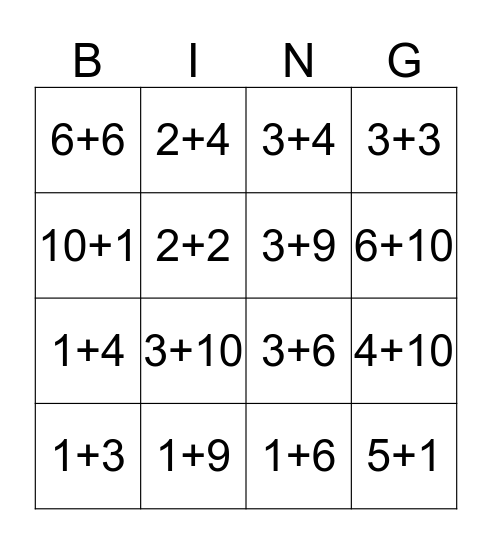# Untitled BingoThis bingo card has 54 words: Free!, 5+1, 8+1, 7+3, 4+6, 4+4, 3+3, 1+1, 2+2, 5+5, 6+6, 7+7, 8+8, 9+9, 10+10, 1+2, 1+3, 1+4, 1+5, 1+6, 1+7, 1+8, 1+9, 10+1, 2+3, 2+4, 2+5, 2+6, 2+7, 2+8, 2+9, 2+10, 3+1, 3+2, 3+4, 3+5, 3+6, 3+7, 3+8, 3+9, 3+10, 4+5, 5+6, 6+7, 7+5, 8+9, 8+10, 7+10, 6+10, 4+10, 3+10, 5+10, 7+9 and 8+9.

⚠ This card has duplicate items: 3+10 (2), 8+9 (2)

## Play Online